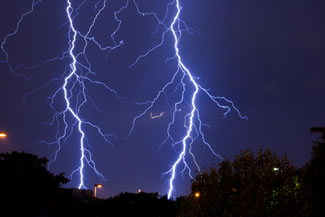#Electricity and Magnetism

Electromagnetism is one of the four fundamental forces of Nature.  The others include:

• the weak nuclear force, the one which binds all known particles in the Standard Model and is responsible for certain forms of radioactive decay.  (In particle physics though, the electroweak interaction is the unified description of two of the four known fundamental interactions of nature: electromagnetism and the weak interaction.)
• the strong nuclear force, which binds quarks to form nucleons, and binds nucleons to form nuclei and

### Revisiting the Millikan Experiment – What’s in an Oil Drop?

All other forces are derived from these fundamental forces and the momentum carried by the movement of particles.

Yet, prior to the unification work carried out  in the 19th century by Michael Faraday and James Clerk Maxwell, electricity and magnetism are thought to be entirely separate forces.

## Maxwell’s Equations of Electromagnetism

In 1864, Maxwell distills the already known properties of electric and magnetic fields into his eponymous set of four equations.

This leads to one of the greatest scientific discoveries, namely the realisation that light is an electromagnetic wave.  At the exception of gravity, electromagnetism is the one fundamental force that is responsible for practically all the natural phenomena encountered daily life above the nuclear scale.

Light propagation, the blueness of the sky, the sparkling of the ocean spray, red sunsets.  Everything.  From the colours of rainbows to the glimmer of butterfly wings, everything can be explained by Maxwell’s equations of Electromagnetism…

Just 4 fundamental equations are all it takes!

## The Four Equations of Electromagnetism$\bigtriangledown \centerdot \boldsymbol{E}=\frac{ \rho}{ \epsilon_{0}}$$\bigtriangledown \centerdot \boldsymbol{B}=0$$\bigtriangledown \times \boldsymbol{E}=-\frac{\partial \boldsymbol{B}}{\partial t}$$\bigtriangledown \times \boldsymbol{B}=\mu_{0} \boldsymbol{J}+ \mu_{0}\epsilon_{0} \frac{\partial \boldsymbol{E}}{\partial t}$

Well, almost…

## Little 'Bytes' about Natural Phenomena, Theoretical Physics and the Latest Worldwide Scientific Findings. Edited from Glasgow, Scotland.